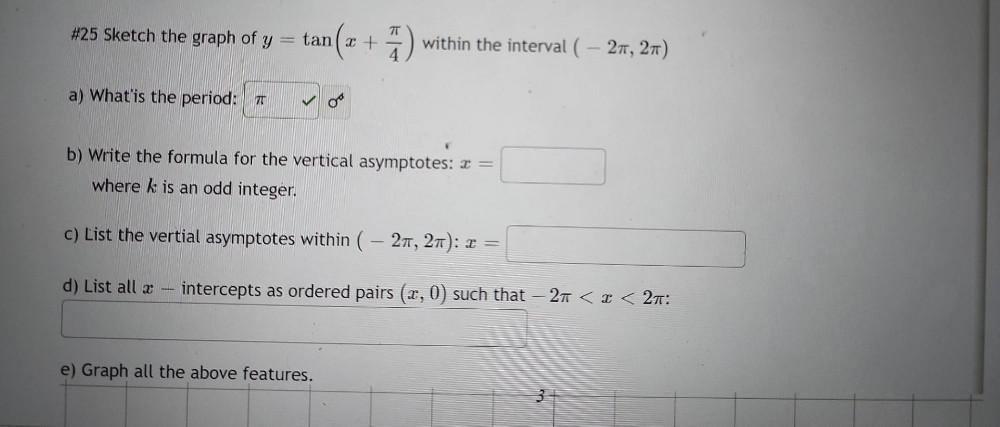Question:

# Sketch the graph of y = tan( x + π/4 ) within the interval ( – 2π, 2π) a) What is the period? b) Write the formula for the vertical asymptotes: x = where k is an odd integer. c) List the verticalSketch the graph of y = tan( x + π/4 ) within the interval ( – 2π, 2π) a) What is the period? b) Write the formula for the vertical asymptotes: x = where k is an odd integer. c) List the vertical asymptotes within ( - 2π, 2π ): x = d) List all x intercepts as ordered pairs ( x,0) such that – 2π < x < 2π: e) Graph all the above features.